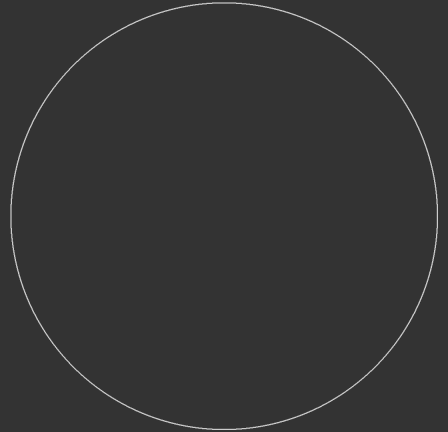→  front page / sitemap / previous page (rectangular mapping)   Search for conformal mapping from Riemann sphere to horn torus and directly from complex plane to horn torus  (considerations are not associated with the dynamic model)   Bend a half longitude continuously to a circle, preserving its length, as shown in the figure below for two opposite ones (treat all half longitudes in this manner simultaneously and recognize that they pass through a series of different spindle tori). The finally obtained cross section of the horn torus bulge has doubled the original angles (from π to 2π), so conclude (fig. 2): to project a point P' from the Riemann sphere onto the horn torus first double the angle α between closer pole (here N) and the point's radius, then draw the perpendicular line from the horn torus bulge (!) center M to this leg of the doubled angle and intersect with horn torus (choose the intersection P" on the same 'hemisphere' on which the original point P' is located on the Riemann sphere). It is the same point as the intersection with the original radius! That was submitted by Vyacheslav Puha already. Now consider and compare the lengths of corresponding latitudes on Riemann sphere and on horn torus (see below): on sphere we have π·sin(α), on horn torus  π·sin2(α), the ratio of both (horn torus : sphere) thus is sin(α), i.e.: length of latitude φ decreases faster than angles α and φ, what means that this projected point P" is not the correct one, that we look for on the horntorus longitude. Unfortunately a stereographic projection P' ↔ P, equivalent α ↔ φ, is not in sight, most likely not possible, and I do not attempt to square the circle, but at least I will offer an analytically generated solution  ...
 click animation to stopSN  = 1/2  (N is 'north pole', S is center, not south pole) P'Q' = 1/2 · sin(α) P"M" = 1/4 · cos(2α) P"Q" = 1/4 · (1 - cos(2α)) length of latitude through P': 2π · P'Q' = π · sin(α) length of latitude through P": 2π · P"Q" = π/2 · (1 - cos(2α)) = π · sin2(α)  Z is the intersection of thestereographic projection linefrom north pole N through point P'with the complex plane, tangent in south pole 0 of Riemann sphere  For compilation we better choosethe version with α = 0 at south pole:continue  ⇒⇒